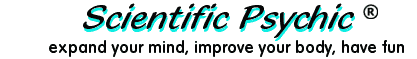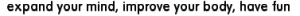Index# Fibonacci's problem of the birdsLeonardo Fibonacci was an Italian mathematician who lived during the Middle Ages (ca. 1170-1240). He is famous for the sequence consisting of the sum of the previous two numbers (1,1,2,3,5,8,13,...). Fibonacci influenced European commerce by introducing the principles of Indian and Arabian mathematics in his book Liber abaci (1202).

Fibonacci posed the following problem in his book:

A man buys 30 live birds that include partridges, pigeons and sparrows for 30 dinars. A partridge costs 3 dinars, a pigeon costs 2 dinars, and two sparrows cost one dinar (i.e., each sparrow costs 1/2 dinar). How many birds of each kind did he buy?

Solution:
Using algebra, we can set up the following equations, where X is the number of partridges, Y is the number of pigeons, and Z is the number of sparrows.

X + Y + Z = 30  (the number of birds equals 30)
3X + 2Y + (1/2)Z = 30  (the total price equals 30)

The problem appears impossible because there are three unknowns but only two equations. However, the statement of the problem has additional ínformation that makes it possible to solve it.

We start by doubling every term of the second equation..

X + Y + Z = 30
6X + 4Y + Z = 60

Then, we subtract the first equation from the second to eliminate Z.

5X + 3Y = 30

From the statement of the problem we know that the three unknowns must all be positive whole numbers because the birds are alive and there are no fractional birds. Notice also in the last equation, that Y cannot be 10 or higher because that would cause X to be zero or negative. Thus, Y has to be an integer between 1 and 9, and in order to isolate X, all the terms of the equation, including 3Y, must be evenly divisible by 5. Looking at all the products of 3 times the integers 1 through 9 (3, 6, 9, 12, 15, 18, 21, 24, 27), we find that only 15 is divisible by 5. Therefore, Y = 5. It follows that X = 3 and Z = 22.

The man bought 3 partridges, 5 pigeons, and 22 sparrows for 30 dinars.